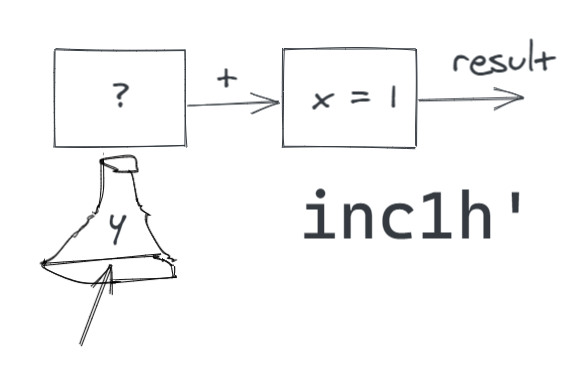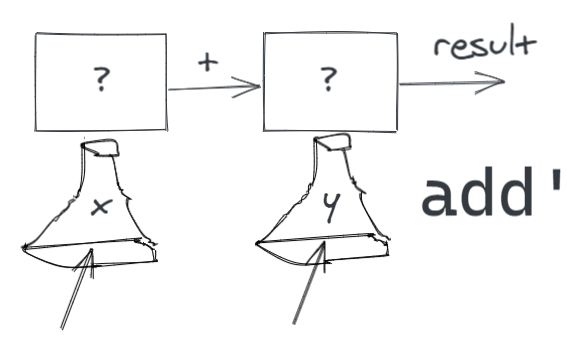# Why FP Matters - Part 2 🎈Picture by Nicky Osipova

Higher-order Functions

# A Quick Recap

In the last article Why FP Matters - Part 1.5 💫: A bit of Haskell, we learned about small bits of Haskell. More specifically we covered the below so that we have a smooth transition into the code used in the white paper - Why Functional Programming Matters by John Hughes1

1. Functions
2. Operators as Functions
3. Pattern Matching
4. Prepend Operator
5. Lists
6. Deconstruction

Before we get into the main event, we need to understand first-class functions.

If you don't know what I am talking about, this is a series of articles. You might want to go here for better context - Why FP Matters - Part 1 🐘 : A brief introduction

# 🥇 First-Class!Picture by Lanty

Functions are first-class citizens of the functional world. What does that mean? I got this definition from the folks that taught me a thing or two about programming and handling complexity.

They may be named by variables. They may be passed as arguments to procedures. They may be returned as the results of procedures. They may be included in data structures.

This feature leads to interesting patterns and it is a new kind of glue which we talked about in the earlier post. It is taken for granted in JavaScript where a function can be passed as a parameter. C# had to include the `Func` keyword for it. Functional languages are built in a manner, making it seamless to work with functions like this.

The LISP family of languages take it further - there is no distinction between function and data.

Examples

So if `f` was a function in Haskell, we could do the below

Here,

• `f` is an identity function.
• It takes in the parameter `x`.
• It returns the value of `x` as is, simple.
`f x = xf 10 -- evaluates to 10`

They may be named by variables.

• Here `g` is assigned to `f`
• `g` can replace `f` anywhere
`g = fg 10 -- evaluates to 10`

They may be passed as arguments.

• Function `f` can be passed to `dof` as an argument
• It will be applied on the other argument `a`
`dof g a = g adof f 10 -- evaluates to 10`

Returned as the results of procedures.

• Here calling `g`, would return `f`
• `x` is ignored.
`g x = fs = g 1s 10 -- evaluates to 10`

May be included in data structures.

• Example: List of functions
`fs = [f, f]`

# 🎈 Higher-order functionsPicture by Morgan Von Gunten

This is common these days and very much possible in most popular languages. Two of the properties from above that are necessary for a language support higher-order functions.

A function is a higher-order function if it satisfies any one or both of the below conditions

• It takes in a function as a parameter
• It should return a function as a result

Let's look at a simple example to clarify this. Let's go back to our `add'` function that we saw in the last post. It is a simple function that takes in two parameters `x` and `y`, then returns the result of adding them.

`add' x y = x + y`

Now say, we want to define several functions say `inc1'`, `inc2'`, `inc3'` and so on, so that these functions take in an input and increment it by `1`, `2`, and `3` respectively.

`inc1' 1 -- evaluates to 2inc2' 1 -- evaluates to 3inc3' 1 -- evaluates to 4`

Without higher-order functions, we would define these functions as below.

`inc1' x = add' 1 xinc2' x = add' 2 xinc3' x = add' 3 x`

🧙🏽‍♂️ "xxxtremely verbose!" ... "you're back?".

Well, I left out one bit of magic from the last post. Let me explain. You see we can change the above code to the below.

`inc1' = add' 1inc2' = add' 2inc3' = add' 3`

# 🌗 Partial ApplicationPicture by Mark Tegethoff

`add' x y = x + y`

You see, when we define the `add'` function as above, Haskell makes it possible for us to provide just one parameter and not the second. There are two ways that you could model this in your head.

Partial Application - Mental Model 1: Incomplete expression

`add' 1` or `add' 2` or `add' 3`, these are incomplete expressions, which are waiting on the second parameter. This hung state of the expression can be assigned to a variable, in our case `inc1'`, `inc2'`, and `inc3'` respectively.

Now when we pass input to an of the `inc*` functions, it passes it through to the waiting expression and completes it. When the expression is complete, it can be fully evaluated to return us a value.

`-- so sinceinc1' = add' 1-- henceinc1' 1--becomesadd' 1 1-- which is evaluated to2`

Note: Each time we call `inc1` on a number, it will run a new instance of that expression. This makes it a reusable expression and it is not exhausted when we use it the first time.

Partial Application - Mental Model 2: Higher-order functions

Below the hood, the incomplete expression can be implemented using higher-order functions and closures. Let's have a look.

How can we return a function from a function? Two ways ...

1. You can return a function from the global scope, we saw the example above `g x = f`
2. You can create a function within a function scope and return it

The second one needs us to create something known as lambdas (λ) or an anonymous function. In the below function, `addh'` returns a function that takes in a parameter `y` and returns the results of `x + y`. Here, `x` is captured from the outer scope and hence we can say that we enclosed over it, or simply that we created a closure.

`-- when addh' is called with a value-- returns a λ capturing the valueaddh' x = \y -> x + yaddh' 1 2 -- evaluates to 3-- when addh' is called with value 1-- returns a λ capturing the value 1-- below is equivalent to \y -> 1 + yinc1h' = addh' 1 inc1h' 2 -- evaluates to 3`We don't have to worry about this, we can stick to the mental model of a hung expression for now. This was just to let you know that Haskell does this by default whenever you define a function. It applies even if a function has three parameters.

`add3' x y x = x + y + zadd3' 1 2 3 -- evaluates to 6add2' = add3' 0add2' 2 3 -- evaluates to 5inc1' = add2' 1inc1' 2 -- evaluates to 3`

# 🥣 CurryingPicture by Jason Leung

If you are still interested, Haskell is named after Haskell Curry, so Haskell does currying, by default 🙂.

So when we define `add'` as below

`add' x y = x + y`

Behind the scenes, Haskell represents `add'` as

`add' :: Num a =>  a ->  a -> aadd' =           \x -> \y ->  x  + y`i.e. it creates

• a lambda
• that takes in `x` and
• returns a lambda
• that takes in `y` and
• returns x + y

If we had three parameters, it would be three lambdas.

Note: `add' :: Num a => a -> a -> a` is the type signature of the method `add'`. We do not have to specify it. Haskell is good at implicitly recognizing this.

• it says that we deal with a generic type `a`
• that the type `a` should be a `Num` because we used a `+` operator
• `a -> a -> a` this part corresponds to the lambdas we talked about. We read the expressions from left to right, `a` goes in, then another `a` goes in and an `a` comes out.

If you leave out the automatic business, we can achieve currying and partial application in some way in any language that supports that treats functions as first-class citizens. This is a small basic step, but most critical.

# Recap

1. We learned about what it means for functions to be first-class.
2. We were introduced to Partial application.
3. Lastly, we tasted some curry.

If you are interested in dabbling around with it you have the below options.

If you don't want to get your hands dirty, that's fine too. We'll continue here with the next one in this series - Why FP Matters.

# Up Next

Why FP Matters - Part 2½ 🍰 - Slicing complexity with Higher-order Functions We will apply our first type of glue that is higher-order functions. We will write small and simple generic parts to process lists. It will be a little domain-specific language.

We will also see examples in Haskell, Scala, JavaScript, and other languages!

For more fun stuff like this, click here to see a list of all my posts

1. Why Functional Programming Matters - http://www.cse.chalmers.se/~rjmh/Papers/whyfp.html